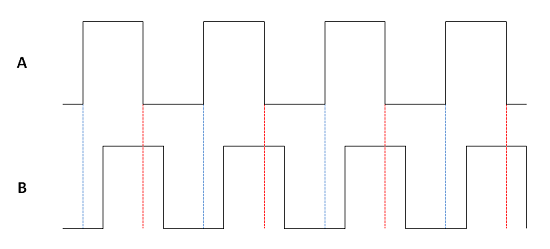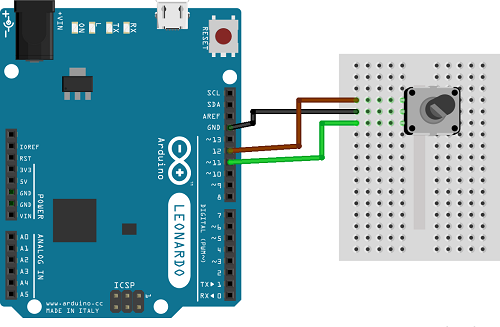# Rotary Encoder 2

## Introduction

This page contains further details about rotary encoders and an alternative (and simpler) way to wire and use an encoder with an Arduino.

The cheaper rotary encoders have 3 pins, usually labelled A, B & C. In the last page we looked at the Sparkfun illuminated encoder - this code should work with any of the basic models that have this 3 pin setup.

A rotary encoder is used to detect rotation. It doesn't supply the position of the encoder, just the details of any change. The following diagram shows what happens to the 2 outputs, A and B as the encoder is rotated. The signals are shown as 'square waves', notice the abrupt changes from low to high - this is a digital output.The two waves are out of phase by 90° (not quite right in my diagram, I know). The dotted red line shows us that, when turning clockwise, and the signal from A changes from high to low, the B signal will be a high. When turning anticlockwise, when A changes from high to low, there is a dotted blue line showing us that the B signal will be low.

So, what matters to us is the change in output on pin A. When that occurs, we read the signal on pin B and can use that to determine the direction in which the encoder was rotated.

## You Will Need

• Rotary Encoder
• Jumper Wires

Like most digital inputs, we need pullup resistors for the A and B pins of the encoder. The Arduino's internal pullups will be used for this project.

## Making The Circuit

The simplest bit of wiring you could do - pin C goes to GND. Pin A is connected to pin 12 and Pin B to pin 11 on the Arduino.## Programming The Arduino

The sketch takes readings from the rotary encoder and outputs the value to the Serial port when there is a change in the reading. Readings are taken every 5 milliseconds. This gives enough time to avoid any false readings. When I use this sketch, I can get all of the values in the range -12 to 12. In fact, this range was deliberately chosen because the rotary encoder I was using has 24 positions - it takes one 360° turn to cover the whole range of my counter.

```// Setting up the counter int reading = 0; int lowest = -12; int highest = 12; int changeamnt = 1; // Timing for polling the encoder unsigned long currentTime; unsigned long lastTime; // Pin definitions const int pinA = 12; const int pinB = 11; // Storing the readings boolean encA; boolean encB; boolean lastA = false; void setup() {   // set the two pins as inputs with internal pullups   pinMode(pinA, INPUT_PULLUP);   pinMode(pinB, INPUT_PULLUP);   // Set up the timing of the polling   currentTime = millis();   lastTime = currentTime;    // Start the serial monitor for debugging   Serial.begin(9600); }  void loop() {   // Read elapsed time   currentTime = millis();   // Check if it's time to read   if(currentTime >= (lastTime + 5))   {     // read the two pins     encA = digitalRead(pinA);     encB = digitalRead(pinB);     // check if A has gone from high to low     if ((!encA) && (lastA))     {       // check if B is high       if (encB)       {         // clockwise         if (reading + changeamnt <= highest)         {           reading = reading + changeamnt;          }       }       else       {         // anti-clockwise         if (reading - changeamnt >= lowest)         {           reading = reading - changeamnt;          }       }       // Output reading for debugging       Serial.println(reading);     }     // store reading of A and millis for next loop     lastA = encA;     lastTime = currentTime;   } }```

## Challenges

Clearly, changing the counter is a good first step. If you can adapt this code to change the way the counter works, then you can use the value of the reading for some other purpose - like fading an LED.

A more interesting project might be to work only with the changes in value. Instead of keeping track of a counter, do something on each change. For example, you could send a left or right keystroke each time the encoder was turned.